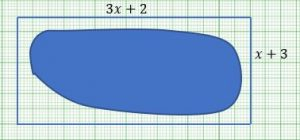1. John plans to get the minimum possible Diamond Razor Mesh fencing material, as a security measure to discourage entry into his dam. He finds out that the rectangular shape drawn around the dam picture can help.  The dimensions are given in algebraic form, in metres. Find an expression for the area of this rectangle.
2. If the area of the rectangle is 66 m2 , form an equation in x.
3. Transform your equation into the form ax2 +bx + c = 0. State the values of a, b and c.
4. What is the possible value of x for which John can afford to buy the necessary fence needed? [Hint: solve the quadratic equation].
5. Find the length and width of the rectangular shape surrounding the dam.
6. Find the total perimeter of the fence needed, if he was to fence right round.
7. John intends to reserve three (3) metres for putting a gate. What is the actual length of the fencing material that John should buy?
8. Diamond Razor Mesh fencing material costs R5000.00 for 30 metres. Find the cost of the actual length of fencing material needed?